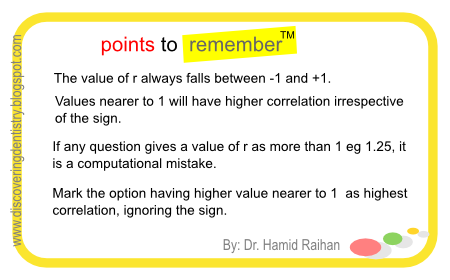## Monday, June 8, 2009

### Correlation Between Variables

Q. Which of the following shows greatest correlation between variables ?
1. r= + 0.25
2. r= + 0.55
3. r= - 0.85
4. r= + 1.25
Answer is 3. r= - 0.85

The correlation in option no. 3 is very high, though it is negative correlation.Print This Document For Studying